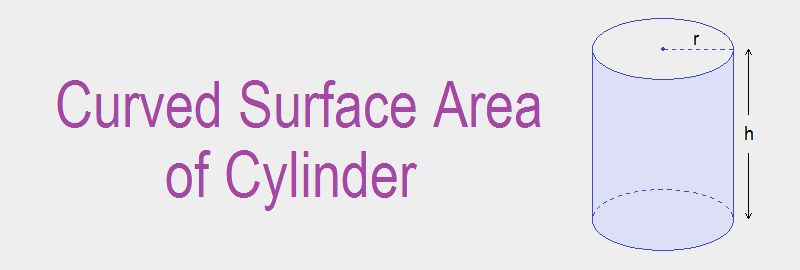# Curved Surface Area of Cylinder## Curved Surface Area of Cylinder

The total area around the curved part of a cylinder is called the curved surface area (CSA) of the cylinder. The curved surface area (CSA) of a cylinder is given by the product of its circumference of circular base (2πr) and height (h) of the cylinder i.e. CSA = 2πrh

This formula can be understood and derived by the following activities.

Let, the cylinder be hollow and made up of thin sheet of paper (or metal). If we cut the curved surface along the height AB and unfold it, then the thin sheet takes the shape of a rectangle ABCD.

The segment DC is coincident with the segment AB when the sheet was not unfolded. The length of AB = h, the height of the cylinder. The length of AD = circumference of the upper circular end = 2πr.

Hence, the curved surface area (CSA) of the cylinder = area of the rectangle ABCD
= 2πr × h
= 2πrh

Curved surface area (CSA) of a cylinder = 2πrh

### Workout Examples

Example 1: Find the curved surface area of the given cylinder.

Solution: Here,
Diameter of the cylinder (d) = 14cm
Radius of the cylinder (r) = 14cm/2 = 7cm
Height of the cylinder (h) = 20cm

We know,
Curved surface area (CSA) = 2πrh
= 2 × 22/7 ×7 ×20
= 880cm2

Thus, the curved surface area of cylinder is 880cm2.

Example 2: The height of a cylinder is 15cm. The curved surface area is 660cm2. Find the radius of the base.

Solution: Here,
Height of the cylinder (h) = 15cm

Curved surface area (CSA) = 660cm2
i.e.     2πrh = 660
or,      2 × 22/7 × r × 15 = 660
or,      660/7 × r = 660
or,      r = 660 × 7/660
or,      r = 7cm

Thus, the radius of the base is 7cm.

Example 3: The curved surface area of a right circular cylinder is 4400cm2 and the circumference of its base is 110cm. Find the height of the cylinder.

Solution: Here,
Circumference of the cylinder = 110cm
i.e.     2πr = 110cm.

Curved surface area  of the cylinder = 4400cm2
i.e.     2πrh = 4400
or,     110h = 4400
or,     h = 4400/110
or,     h = 40cm

Thus, the height of the cylinder is 40cm.

You can comment your questions or problems regarding the CSA of cylinder here.